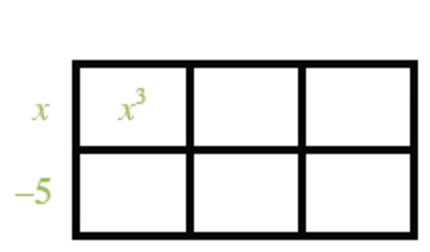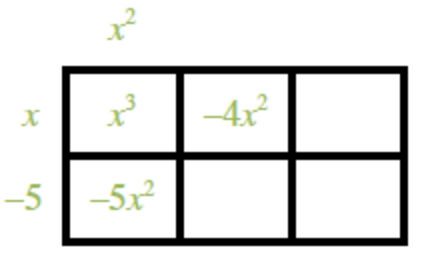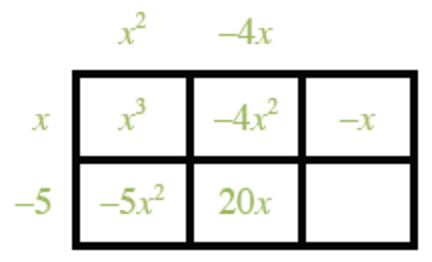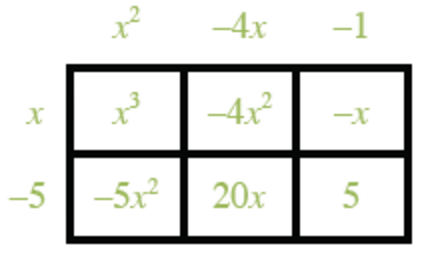### Home > INT3 > Chapter 8 > Lesson 8.3.1 > Problem8-100

8-100.

Now divide x3 – 9x2 + 19x + 5 by the factor that you chose in the previous problem. If it is a factor, use it and the resulting factor to determine all the roots of the polynomial. If it is not a factor, reconsider your answer to the previous problem and try a different factor. Homework Help ✎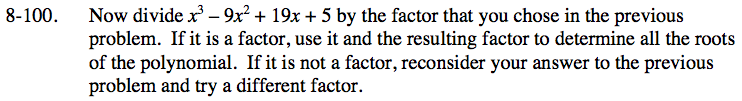x3 − 9x2 + 19x + 5 = (x − 5)(x2 − 4x − 1)

x − 5 = 0
x2 − 4x − 1 = 0

Now finish solving.